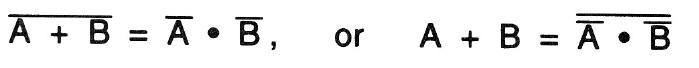De Morgan's theorem
Electronic

74HC00, 74HC02Have you ever heard of De Morgan's theorem?

It's one of the basic theorems we encounter in logical mathematics. In this project, we're going to prove this theorem which is expressed as follows.Not sure what this means?

Well, in ordinary language, this can be expressed as The OR gate can be constructed by an AND gate and an inverter. De Morgan's theorem says that the gate circuit (U1) for LED 1 and the gate circuit (U2) for LED 2 are logically equal.

Let's check this by turning power ON after wiring the project. Use S1 and S2 to see that the lighting statues of LED 1 and LED 2 match with each other. De Morgan's theorem also tells us the following formula.Recherche personnalisée